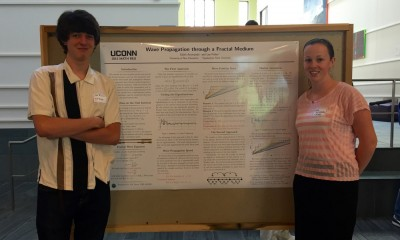# Power Dissipation in Fractal AC Circuits

## Supervisors

Alexander TeplyaevLuke Rogers, Antoni Brzoska, Ulysses Andrews

## Overview

In this project, Feynman’s analysis of an infinite ladder circuit is extended to fractal circuits, in particular, a Sierpinski Ladder circuit and two variants of a Hanoi circuit.   These circuits are constructed by taking the limit of graph approximations with inductors and capacitors placed along edges in a well-defined manner.  Inductors, capacitors and resistors all contribute to the impedance within a circuit; but whereas a resistor imparts a real impedance, inductors and capacitors impart a purely imaginary impedance.

For each circuit, the following was accomplished.  First, the net impedance between certain boundary points of the circuit was computed.  Second, the filter conditions for each circuit were found.  A circuit becomes a filter when the net impedance between boundary vertices has a positive real part!  Third, it was proved that these impedances can be obtained by placing a small positive resistance epsilon on each edge of the graph approximations, finding the limiting impedance between the boundary vertices, and then taking epsilon to zero.  Finally, the construction of harmonic functions on these circuits was outlined.

# arXiv:1605.03890

## Presentation

Fractal AC Circuits

Poster

# Stochastic Stability of Planar Flows

## Publication: “Stabilization by noise of a C^2-valued coupled system.” Joe P. Chen, Lance Ford, Derek Kielty, Rajeshwari Majumdar, Heather McCain, Dylan O’Connell, and Fan Ny Shum. Stochastics and Dynamics Vol. 17, No. 06, 1750046 (2017)

arXiv.org:1510.09221

## Project

Project Information

# Stochastic Stability of Planar Flows

## Publication: arXiv:1510.09221

 Journal reference: Stochastics and Dynamics, Vol. 17, No. 6 (2017) 1750046 DOI: 10.1142/S0219493717500460

## Event

REU Mini-Symposium @ UConn

JMM Poster Session @ Seattle

Poster

## Project

Project Information

# Group Members

Edith Aromando, Lee Fisher## Overview

We consider the wave equation on the unit interval with fractal measure, and use two numerical models to study wave speed and propagation distance.  The first approach uses a Fourier series of eigenfunctions of the fractal Laplacian, while the second uses a Markov chain to model the transmission and reflection of classical waves on an approximation of the fractal. These models have complementary advantages and limitations, and we conjecture that they approximate the same fractal wave.

Poster